Courses

# Chapter 1 (Part 2) AC Bridges - Notes, Electrical Measurement, Electrical Engineering Electrical Engineering (EE) Notes | EduRev

## Electrical Engineering (EE) : Chapter 1 (Part 2) AC Bridges - Notes, Electrical Measurement, Electrical Engineering Electrical Engineering (EE) Notes | EduRev

The document Chapter 1 (Part 2) AC Bridges - Notes, Electrical Measurement, Electrical Engineering Electrical Engineering (EE) Notes | EduRev is a part of the Electrical Engineering (EE) Course Electrical Engineering SSC JE (Technical).
All you need of Electrical Engineering (EE) at this link: Electrical Engineering (EE)

Schering Bridge :

• It is widely used for the measurement of capacitance and dissipation factor (D).
• In particular, it may be used in the measurement of relative permittivitty, properties of insulators, capacitor bushings, insulating oil.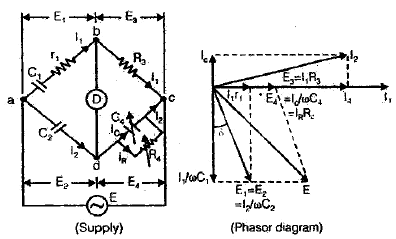Let Cand rare the unknown quantities

C2 = a standard capacitor
C4 = a variable capacitor
R3 = a non-inductive resistance
R= a variable non-inductive resistance

At balance condition,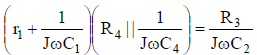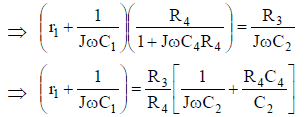Equating real part we get,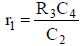Equating imaginary part we get,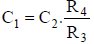• Here two independent balances are obtained if Cand R4 are chosen as the variable elements.

∴ Dissipation Factor = D1 = ωC1r1 = ωC 4R 4

• For the Low value of unknown capacitance “C1” so is Z1 and then detector cannot detected.
• For the detector to be detects the supply frequency “f” should be higher and higher. At this stage, this type of Schering bridge is called “High voltage Schering bridge”.
• Due to “High frequency effect”. “Stray capacitance of Earth” will be generated and so leakage currents are significant so it can not be neglected. Finally there is an error in measurement.
• To eliminate this error, bridge should be balanced in such a  manner that Vb = Vd = 0 i.e. both ‘b’ and ‘d’ should be virtually grounded.
• For this purpose a new arrangement is used and such device is called “Wagner’s Earthing Device”.

AC bridges through which frequency can be measured :

1. Wien’s Bridge

Wien’s Bridge :

• It is primarily well known as a frequency determining bridge.
• It may be employed in a “Harmonic distortion analyzer” where it is used as “Notch filter”.
• There also finds applications in Audio and High frequency oscillators as the frequency determining device (100 Hz-100 KHz).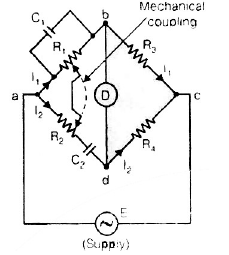At balance condition, Zab . Zcd = Zad . Zbc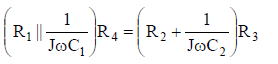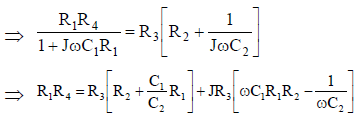Equating real part we get,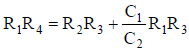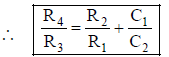Equating imaginary part wet get,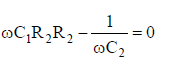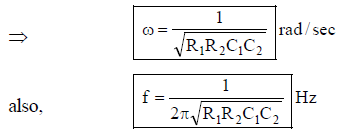In most of the Wien’s bridges, R1 = R2 = R and C= C2 = C

then, equation becomes,

R4 = 2R3

and equation becomes,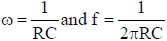• The bridge may be used in “Frequency determining device” balanced by a single control and this control may be calibrated directly in terms of frequency.
• It may also be used for the measurement of “Capacitance”.

Measurement of Resistance :

Classification of resistance

Low resistance ⇒ R <
Medium resistance ⇒ 1Ω < R < 0.1M Ω
High resistance ⇒ R > 0.1 M Ω

Measurement of Medium Resistance

The different Methods used for measurement of medium resistance are:

(i) Voltmeter-Ammeter method
(ii) Substitution method
(iii) Wheatstone bridge method
(iv) Ohmeter method

Voltmeter-Ammeter Method:

• This method is very popular since the instruments required for this test are usually available in the laboratory.
• Measured value of resistance is given by: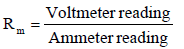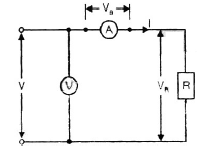Let Ra be the resistance of the ammeter.

∴ Voltage across the ammeter , Va = IRa

Now measured value of resistance,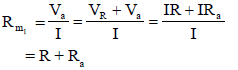∴ True value of resistance,

R = Rm1 - Ra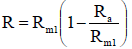• Thus the measured value of resistance is higher the value. It is also clear from above that the true value is equal to measured value only when the ammeter resistance, Ra is zero.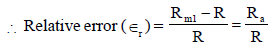• It is clear from equation (4.40) that the error in measurements would be small if the value of resistance under measurement is large as compared to the internal resistance of the ammeter.
• So the type of circuit should be used when measuring high resistance values.

Ammeter voltmeter method

• Voltmeter connected near the load.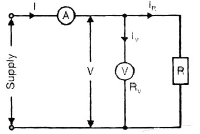• In this circuit the voltmeter measures the true value of voltage but the ammeter measures the sum of currents through the resistance R and the voltmeter V.

I = IR + IV

Let RV be the resistance of the voltmeter.

∴ Current through the voltmeter.

Iv = V/Rv

Measured value of resistance,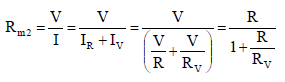Now, true value of resistance is: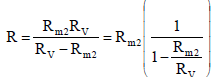• From this equation, it is clear that the true value of resistance is the measured value only if the resistance of voltmeter Rv, is infinite however, if the resistance of voltmeter is very large as compared to the resistance under measurement.

or, RV >> Rm2, and therefore Rm2/ Rv  is very small.

Now equation may be written as,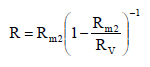By binomial theorem,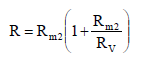⇒Thus the measured value of resistance is smaller than the true value.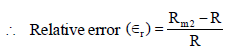from equation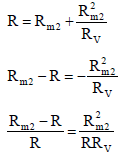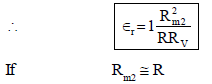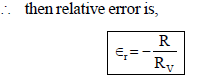Note: ⇒ This method is used when measuring low resistance values.

• Substitution Method :  Substitution method is more accurate method than the ammeter voltmeter method, as it is not subjected to the errors encountered in this method.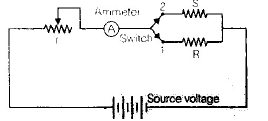Let, R = unknown resistance

S = standard variable resistance

r = regulating resistance and there is a switch for putting R and S into the circuit alternately.

Operation

• The switch is put at position 1, and resistance R is connected in the circuit. The regulating resistance r is adjusted till the ammeter pointer is at a chosen scale mark.
• Now the switch is thrown to position 2. Putting the standard variable resistance S in the circuit. The value of S is varied till the same deflection, as was obtained with R in the circuit, is obtained. The settings of the dials of S are read.
• Thus the value of unknown resistance R is equal to the dial settings of resistance S,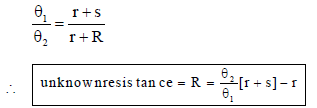where, θ1 = the deflection with standard resistor.

θ= the deflection with unknown resistor in circuit.

G is the galvanometer.

Wheatstone Bridge Method :

• The Wheatstone bridge is an instrument for making comparison measurements and operates upon a null deflection principle.
• A very important device used in the measurement of medium resistance is the Wheatsone bridge.
• It is highly accurate and reliable instruments, because the indication is independent of the calibration of the null indicating instrument or any of its characteristics.
• This method is extensively used in industries.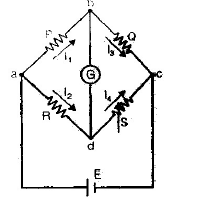where, R is the unknown resistance.

S is called the ‘standard arm’ or known resistance of the bridge.

and P and Q are called the ratio arms for bridge balance, we can write

I1P = I2R

I1 / I2 = R/P

for the galvanometer current to be zero, The following conditions also exist: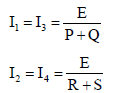where, E = emf of the battery. From the equation;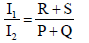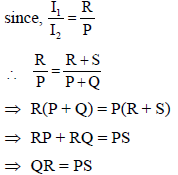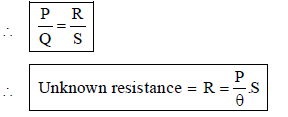Carey-Foster Slide-wire Bridge :  This method is used for the purpose of determining the difference between the standard and the unknown resistances.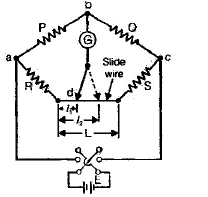• Exact balance is obtained by adjustment of the sliding contact on the ide-wire.

Let l1 be the distance of the sliding contact from the left-hand end of the slide wire. The resistances R and S are then inter-changed and balance is again obtained. Let the distance now be l2.
Ist case : For the Ist balance,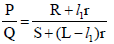Where r is the resistance per unit length of the slide wire.
IInd case : For the 2nd balance,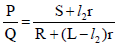where L is length being included between R and S.
Now, from equation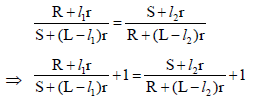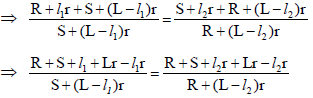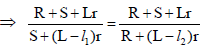Hence,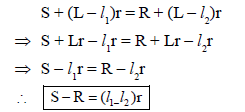Ohmmeter method
• Ohm-meter method is used for measuring resistance of field coils of machines.
• It is used for measurement of heating element resistance.
• It is also used in measuring and sorting of resistors.

Measurement of Low Resistance :
• Construction : These are provided with four terminal to eliminate the effect of contact resistance. Out of four terminals, two terminals are used for current injection, these are current terminals and remaining two terminals are used for measurement of potential dropped across the resistances. These terminals are called voltage terminals.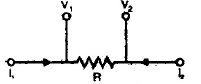Here, I1 and I2 are current terminals V1 and V2 are voltage terminals.

Methods for measurement of low resistance
• Ammeter-voltmeter method.
• Kelvin’s double bridge method.
• Potentiometer method

⇒ Ammeter-voltmeter methods are already discussed in this chapter previously.

Kelvin’s Double Bridge Method for Measurement of Low Resistances :
• The Kelvin’s bridge is a modification of the Wheatstone bridge and provides greatly increased accuracy in measurement of low value resistances.
• The Kelvin’s bridge arrangement are given in figure below,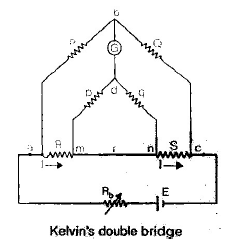• The first set of ratio arms is P and Q. The second set is of ratio arms, P and q which is used to connect the galvanometer to point d at the appropriate potential between points m and n to eliminate the effect of connecting lead of resistance r between the known resistance, R and standard resistance S.
• The ratio p/q is made equal to P/Q. Under balance conditions there is no-current through the galvanometer, the voltage drop between a and b i.e. Eab is equal to voltage drop Eamd between a and c.
Now,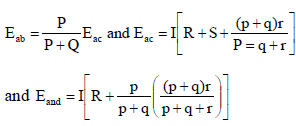for zero galvanometer deflection, Eab = Eamd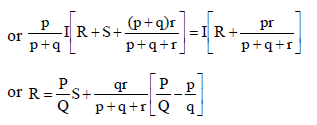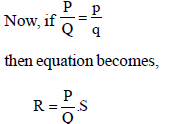Note:

⇒ This equation shows the usual working equation for the Kelvin bridge.
⇒ It indicates that the resistance of connecting lead, r1 has no-effect on the measurement, provided that the two sets of ratio arms has equal ratios.

Potentiometer Method :
• This method is a comparison type method. Measurement using comparison methods are capable of a high degree of accuracy because the result obtained does not depend upon on the actual deflection of a pointer, as is the case in deflectional methods, but only upon the accuracy with which the voltage of the reference source is known.

Measurement of High Resistance :
• The different methods employed are :

• Direct deflection method.
• Loss of charge method.
• Megaohm bridge method.
• Meggar method.

• High resistance of the order of hundreds or thousands of megaohm are often encountered in electrical equipment, and frequently must be measured.

• Common examples are :

• Insulation resistance of components and built up electrical equipment like machines and cables.
• Leakage resistance of capacitors.
• Volume resistivity of a material.
• Surface resistivity.
• Resistance of high resistance circuit elements like in vaccun tube circuits.

Difficulties in Measuring High Resistances :
• Error due to leakage current.
• Error due to electrostatic effect or charges are gathered.
• Error due to capacitive effect.

Note :
⇒ Problem of leakage current can be eliminated by using guard circuit.
⇒ In loss of charge method, on the principle of discharging of capacitor through unknown resistance, for high resistance value.
⇒ Meggar method is used for measurement of insulation resistance and earth resistance.

Offer running on EduRev: Apply code STAYHOME200 to get INR 200 off on our premium plan EduRev Infinity!

65 docs|37 tests

,

,

,

,

,

,

,

,

,

,

,

,

,

,

,

,

,

,

,

,

,

,

,

,

,

,

,

;